# Metric geometry : Haz elíptico de circunferencias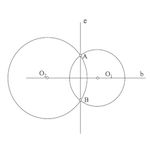When you define a beam circumferences infinity as a set simply fulfilling a restriction based on the power, sorted the beams depending on the relative position of its elements.

Los elliptical beam circumferences These families are among circumferences. We will see how to determine elements that belong.

Given the circles drying on a couple of points, the radical axis "and" of the circumferences coincides with the chord common to both circumferences. This line is perpendicular to the one containing the centers of the circumferences.

The endless circles through a couple of points, determine one elliptical beam circumferences. The points common to all key points are called beam

The radical axis of any two circles of this bundle is the line and.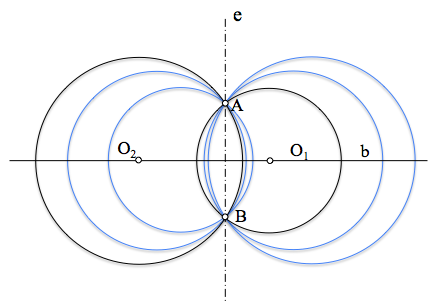All centers of the circumferences of the bundle are in a straight, b, denominada straight beam foundation.

### Determining a circumference of the elliptical beam which passes through a point P

From the endless circles of elliptical beam, only one pass through a given point. Let's see how to determine the center of a circle of the beam passing through a point P any.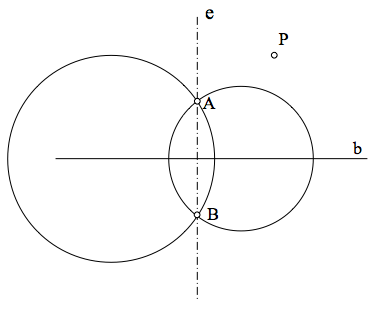The circle will have its center sought O1 based on the straight line, b, and go through the key points A and B, and by P, so shall also the bisector of these points.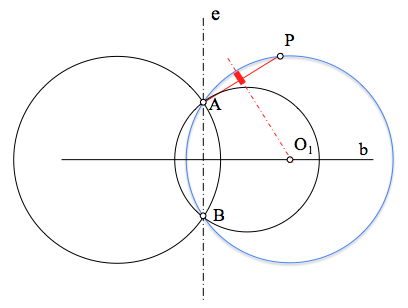Solution, its center, thus determined by the intersection of two loci, the base and the perpendicular line segment AP containing two crossing points.

### Determine the elliptical beam circumferences are tangent to a given line

Tangency condition is determined by a straight t anyone who does not match with right or basis b or the radical axis and. The beam can be defined by its fundamental points A and B can pass all the circles that belong.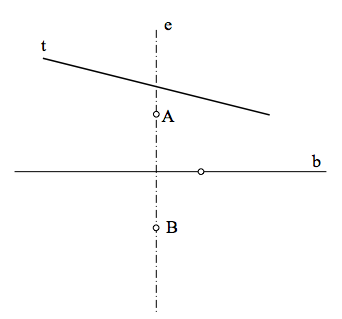To solve the problem look for a point Cr, radical shaft and, have equal power with respect to the beam circumferences, and belonging, and the embroidery, to the line t ya the latter is the center of the circumferences radical which are tangent. We see, that Cr is the radical center of the line t (infinite radius circumference) and parabolic beam circumferences.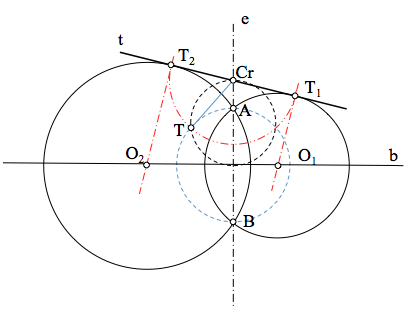As shown in Figure, power Cr on all the circumference of the beam can be determined by finding the tangent (squared) any circumference of the beam (in this case the diameter AB). This distance is to be also the points of tangency of the solutions sought. We have two solutions because we can take this away Cr-O on both sides of Cr on the line t.

### Determine the elliptical beam circumferences are tangent to a given circle

The generalization of the problem comes when the condition of tangency is on a circle t any.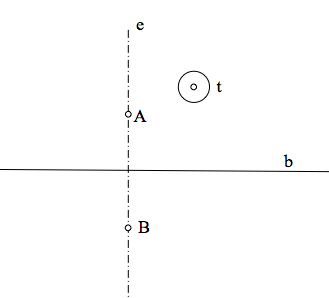In this case, again, determine a point Cr having equal power over the circumference makes the tangency condition and any of the elliptical beam, so it must be in its radical axis.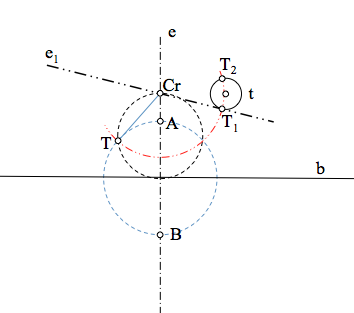The solutions pass through the points T1 and T2 located on tangents drawn from Cr, and which are at the root of the distance power that we have calculated as in the previous case.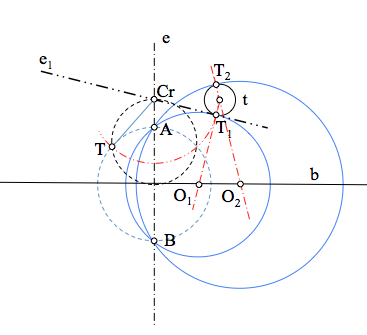The centers of the solutions found aligned with the center of the circle t and corresponding contact points.

## Make conjugate

Last, we can see in the figure below the conjugate beam (orthogonal) of an elliptical beam, that, as discussed later, is another basis hyperbolic straight anterior radical shaft.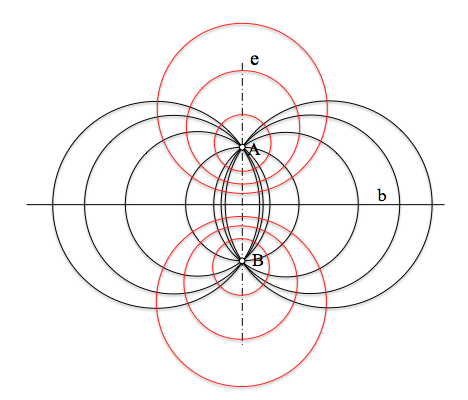Metric geometry